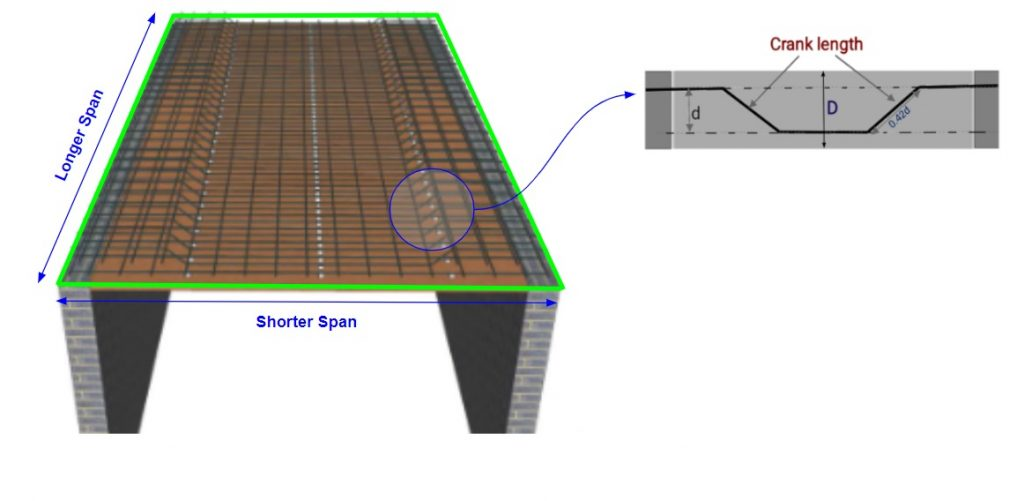Work Hours
Monday to Friday: 7AM - 7PM
Weekend: 10AM - 5PM

# Steel Weight Calculator For One Way Slab

## Calculations for Steel Calculator for One Way Slab

Let’s understand with the help of an example, how to use the above Steel Calculator for Slab. Distribution bars are provided in the longer span of the one-way slab & main bars are placed along the shorter span of the slab.Assumptions for Example

• There are 2 types of bars in one way slab: Main Bar & Distribution Bar
• Main Bar Diameter = 10 mm
• Spacing Between Main Bars(C/C distance) = 150 mm
• Distribution Bar Diameter = 8 mm
• Spacing Between Distribution Bars(C/C distance) = 100 mm
• Length of Slab = 6000 mm
• Width of Slab = 3000 mm
• Width of Beam of Slab = 300
• Concrete Cover = 25 mm
• Slab Thickness = 150 mm
• We are using Steel grade of fe500

Calculation Procedure

• Step 1: Calculation of total no. of bars
Calculate the number of bars required (main and distribution both).
Number of Main Bars = (Length of Longer Side / Spacing) + 1
=(( 6000/150)+1) = 41
Number of Distribution Bars = (Length of Shorter Side / Spacing) + 1
= ((3000/100)+1) = 31

• Step 2: Calculation of cutting length of main & distribution bar
Cutting Length of Main Bar = Clear Span (L) + (2 x Beam Width) – (2 x Con. Cover) + (1 x Inclined Length) – (45° bend x 2)
where L = Clear Span of the Slab ;0.42D = Inclined length (Bend length) ;1d = 45° bends (d is diameter of bar)
First calculate the length of “D“. i.e D = (Thickness) – 2 (Clear cover at Top, Bottom) – Diameter of the bar.
D = 150- 2×25 – 10 = 90 mm
Cutting Length of Main Bar = 3000 – 2×300 – 2×50 + 0.42×90 – 2×10= 2318 mm
Cutting Length of Distribution Bar = Clear Span (Lclear) – (2 x Con. Cover)
= 6000 – 2×25 = 5950 mm

• Step 3: Calculation of Top Distribution Bar
Numbers of Top distribution bar for longer span = {(L/4)/spacing + 1} × 2 sides
= ((3000/4)/100 +1) = 9
Cutting Length of Top Distribution Bar = Clear Span – (2 x Con. Cover)= 5950 mm

• Step 4: Total Length Calculation
Total Length of Main Bar = 41 x 2318 = 95038 mm = 95.038 m
Total Length of Distribution Bar = 31 x 5950 + 9 x 5950 = 238000 mm = 238 m

• Step 5: Calculation of Steel Weight
Weight of the bar as per their length and diameter by following Formula: –
Unit weight formula for kg per metre =(D^2/163) × L kg/m
Unit weight for feet per metre = (D^2 / 533) × L kg/ft
L is the required length of Steel bar of Diameter D
Weight of Main Bar = (102/163) x 95.038 = 58.31 Kg
Weight of Distribution Bar = (82/163) x 238 = 93.45 Kg

Final Result

Total Steel Weight =  58.31 + 93.45 = 151.76 Kg

Try Onsite, #1 Tool For Site Tracking